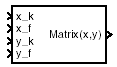# Interpolate Matrix(x,y)

Return interpolated matrix for given inputs

• Library:
• Aerospace Blockset / GNC / Control

•## Description

The Interpolate Matrix(x,y) block interpolates a two-dimensional array of matrices. In two-dimensional cases, the interpolation is carried out first on x and then y. For more information, see Algorithms.

The matrix to be interpolated must be four-dimensional, the first two dimensions corresponding to the matrix at each value of x and y. For example, if you have four matrices A, B, C, and D defined at `(x = 0.0,y = 1.0)`, `(x = 0.0,y = 3.0)`, `(x = 1.0,y = 1.0)` and `(x = 1.0,y = 3.0)`, then the input matrix is given by

`matrix(:,:,1,1) = A;`

`matrix(:,:,1,2) = B;`

`matrix(:,:,2,1) = C;`

`matrix(:,:,2,2) = D;`

## Limitations

This block must be driven from the Prelookup block.

## Ports

### Input

expand all

First interpolation index i, specified as a scalar and vector.

Data Types: `double`

First interpolation fraction λ x, specified as a scalar

Data Types: `double`

Second interpolation index j, specified as a scalar.

Data Types: `double`

Second interpolation fraction λ y, specified as a scalar.

Data Types: `double`

### Output

expand all

Interpolated matrix, specified as a matrix.

Data Types: `double`

## Parameters

expand all

Matrix to be interpolated, with four indices and the third and fourth indices labeling the interpolating values of x and y.

#### Programmatic Use

 Block Parameter: `matrix` Type: character vector Values: matrix Default: `'matrix'`

## Algorithms

This two-dimensional case assumes the matrix is defined as a function of two independent variables, x = [ x1x2x3... xixi+1 ... xn] and y = [ y1y2y3 ... yjyj+1 ... ym]. For given values of x and y, four matrices are interpolated. Then for xi < x < xi+1 and yj < y < yj+1, the output matrix is given by

`$\begin{array}{l}\left(1-{\lambda }_{y}\right)\left[\left(1-{\lambda }_{x}\right)M\left({x}_{i},{y}_{j}\right)+{\lambda }_{x}M\left({x}_{i+1},{y}_{j}\right)\right]+\\ {\lambda }_{y}\left[\left(1-{\lambda }_{x}\right)M\left({x}_{i},{y}_{j+1}\right)+{\lambda }_{x}M\left({x}_{i+1},{y}_{j+1}\right)\right]\end{array}$`

where the two interpolation fractions are denoted by

`${\lambda }_{x}=\left(x-{x}_{i}\right)/\left({x}_{i+1}-{x}_{i}\right)$`

and

`${\lambda }_{y}=\left(y-{y}_{j}\right)/\left({y}_{j+1}-{y}_{j}\right)$`

## Version History

Introduced before R2006a